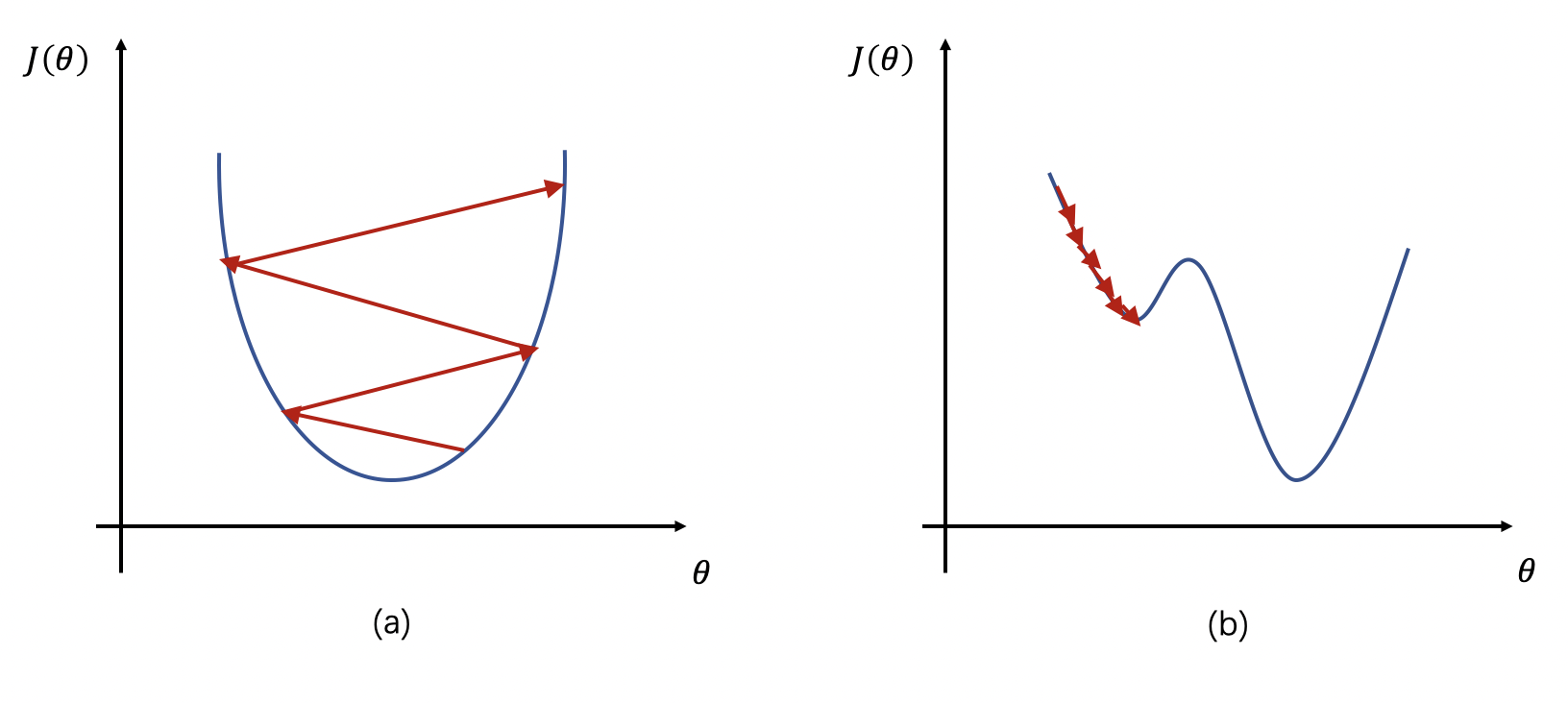# 学习率¶

## 2. 学习率对网络的影响¶## 3. 学习率的设置¶

• 分段常数衰减（Piecewise Decay）

在不同的学习阶段指定不同的学习率，在每段内学习率相同。该过程可以举例说明为：

boundaries = [100, 200]  # 指定学习率改变的边界点为100和200
values = [1.0, 0.5, 0.1] # 指定不同区间下的学习率大小

learning_rate = 1.0  if epoch < 100
learning_rate = 0.5  if 100 <= epoch < 200
learning_rate = 0.1  if epoch >= 200


• 指数衰减（Exponential Decay）

学习率随训练轮数成指数衰减，每次将当前学习率乘以给定的衰减率得到下一个学习率。指数衰减的公式可表示为：

$new\_learning\_rate = last\_learning\_rate * gamma$

其中，$$gamma$$ 为衰减率。

• 自然指数衰减 （Natural Exponential Decay）

每次将当前学习率乘以给定的衰减率的自然指数得到下一个学习率。其公式表达为：

$new\_learning\_rate = learning\_rate * e^{-gamma*epoch}$

其中，$$learning\_rate$$ 为初始学习率，$$gamma$$ 为衰减率，$$epoch$$ 为训练轮数。

• 多项式衰减（Polynomial Decay）

通过多项式衰减函数，学习率从初始值逐渐衰减至最低学习率。其中，参数 $$cycle$$ 代表学习率下降后是否重新上升。若 $$cycle=True$$，则学习率衰减至最低后会重新上升到一定值，再降低至最低学习率并进行循环。若 $$cycle = False$$，则学习率从初始值单调递减至最低值。

$$cycle=True$$，其计算公式为：

\begin{split} \begin{align} decay\_steps &= decay\_steps * math.ceil(\frac{epoch}{decay\_steps}) \\ new\_learning\_rate &= (learning\_rate - end\_lr) * (1 - \frac{epoch}{decay\_steps})^{power} + end\_lr \end{align} \end{split}

$$cycle=False$$，其计算公式为：

\begin{split} \begin{align} epoch &= min(epoch, decay\_steps) \\ new\_learning\_rate &= (learning\_rate - end\_lr) * (1 - \frac{epoch}{decay\_steps})^{power} + end\_lr \end{align} \end{split}

其中，$$learning\_rate$$ 为初始学习率，$$decay\_step$$ 为进行衰减的步长，$$end\_lr$$ 为最低学习率，$$power$$ 为多项式的幂。

• 间隔衰减 （Step Decay）

学习率按照指定的轮数间隔进行衰减，该过程可举例说明为：

learning_rate = 0.5 # 学习率初始值
step_size = 30      # 每训练30个epoch进行一次衰减
gamma = 0.1         # 衰减率

learning_rate = 0.5    if epoch < 30
learning_rate = 0.05   if 30 <= epoch < 60
learning_rate = 0.005  if 60 <= epoch < 90
...

• 多间隔衰减（Multi Step Decay）

学习率按特定间隔进行衰减，与间隔衰减的区别在于：间隔衰减的epoch间隔是单一且固定的，而多间隔衰减中的epoch间隔是预先指定的多间隔。该过程可举例说明为：

learning_rate = 0.5    # 学习率初始值
milestones = [30, 50]  # 指定轮数间隔
gamma = 0.1            # 衰减率

learning_rate = 0.5    if epoch < 30
learning_rate = 0.05   if 30 <= epoch < 50
learning_rate = 0.005  if 50 <= epoch
...

• 逆时间衰减（Inverse Time Decay）

学习率大小与当前衰减次数成反比。其计算公式如下：

$new\_learning\_rate = \frac{learning\_rate}{1 + gamma * epoch}$

其中，$$learning\_rate$$ 为初始学习率，$$gamma$$ 为衰减率，$$epoch$$ 为训练轮数。

• Lambda衰减（Lambda Decay）

使用lambda函数来设置学习率，其中lambda函数通过epoch计算出一个因子，使用该因子乘以初始学习率。该衰减过程可参考如下例子：

learning_rate = 0.5                      # 学习率初始值
lr_lambda = lambda epoch: 0.95 ** epoch  # 定义lambda函数

learning_rate = 0.5                      # 当epoch = 0时，0.5 * 0.95 ** 0 = 0.5
learning_rate = 0.475                    # 当epoch = 1时，0.5 * 0.95 ** 1 = 0.475
learning_rate = 0.45125                  # 当epoch = 2时，0.5 * 0.95 ** 2 = 0.45125
...

• 余弦衰减（Cosine Annealing Decay）

使用 cosine annealing 的策略来动态调整学习率，学习率随step数变化成余弦函数周期变化。该方法为论文 SGDR：Stochastic Gradient Descent with Warm Restartscosine annealing动态学习率。学习率调整公式为：

\begin{split} \begin{align} \eta_t = \eta_{min} + \frac{1}{2}(\eta_{max} - \eta_{min})(1 + cos(\frac{T_{cur}}{T_{max}}\pi)), \quad T_{cur} \neq (2k+1)T_{max} \\ \eta_{t+1} = \eta_{t} + \frac{1}{2}(\eta_{max} - \eta_{min})(1 - cos(\frac{1}{T_{max}}\pi)), \quad T_{cur} = (2k + 1)T_{max} \end{align} \end{split}

其中，$$\eta_{max}$$的初始值为学习率的初始值，$$T_{cur}$$是SGDR训练过程中的当前训练轮数。

• 诺姆衰减（Noam Decay）

诺姆衰减的计算方式如下：

$new\_learning\_rate = learning\_rate * d_{mode}^{-0.5}*min(epoch^{-0.5}, epoch*warmup\_steps^{-1.5})$

其中，$$d_{model}$$ 代表模型的输入、输出向量特征维度，$$warmup\_steps$$ 为预热步数，$$learning\_rate$$ 为初始学习率。更多细节请参考 attention is all you need

• loss自适应衰减（Reduce On Plateau）

当loss停止下降时，降低学习率。其思想是：一旦模型表现不再提升，将学习率降低 2-10 倍对模型的训练往往有益。此外，每降低一次学习率后，将会进入一个冷静期。在冷静期内不会监控loss变化也不会进行衰减。当冷静期结束后，会继续监控loss的上升或下降。

• 线性学习率热身（Linear Warm Up）

线性学习率热身是一种学习率优化策略，在正常调整学习率前，先逐步增大学习率。

当训练步数小于热身步数（warmup_steps）时，学习率 $$lr$$ 按如下方式更新：

$lr = start\_lr + (end\_lr - start\_lr) * \frac{epoch}{warmup\_steps}$

当训练步数大于等于热身步数（warmup_steps）时，学习率 $$lr$$ 为：

$lr = learning\_rate$

其中，$$lr$$ 为热身之后的学习率，$$start\_lr$$ 为学习率初始值，$$end\_lr$$ 为最终学习率，$$epoch$$ 为训练轮数。